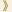HomeShaw Laureates2017Mathematical SciencesPress Release

The Shaw Prize in Mathematical Sciences 2017 is awarded in equal shares to János Kollár, Professor of Mathematics, Princeton University, USA and Claire Voisin, Professor and Chair in Algebraic Geometry, Collège de France, for their remarkable results in many central areas of algebraic geometry, which have transformed the field and led to the solution of long-standing problems that had appeared out of reach.

Since ancient times, a central theme in mathematics has been the study of polynomials and their solutions. Algebraic geometry is the study of the properties of sets of solutions to polynomial equations in several variables. A simple example of such an equation is x2 + y2 + z2 = 1, the solution set of which is the surface of a sphere of radius 1.

As this example demonstrates, solution sets of polynomial equations, which are known as varieties, are geometric objects. Examining the interplay between the algebra and the geometry has turned out to be remarkably fruitful, and algebraic geometry is a major branch of mathematics, the study of which has profound consequences not just for algebra and geometry, but also for several other areas ranging from number theory to mathematical physics.

Two varieties are said to be birationally equivalent if they become the same after excluding a suitably small subset. Rational varieties are those that are birationally equivalent to ordinary n-dimensional space, for some n. Some of the most exciting advances in algebraic geometry over the past few decades have been in better understanding the birational classification of higher-dimensional varieties. For example, Shigefumi Mori won the Fields Medal for his Minimal Model Program. Some of the most important breakthroughs have been in characterizing rational varieties. Both János Kollár and Claire Voisin have made central contributions to this development.

Along with his work on birational classification, Kollár’s most recent work stands out in a direction that will influence algebraic geometry deeply in the decades to come, as an important complement of the Minimal Model Program: the definition and study of moduli of higher-dimensional varieties, which can be thought of as sophisticated geometrical structures whose points represent equivalence classes of these varieties. The importance of this area can be seen in the immense wealth of papers on the moduli problem in dimension 1, which today occupies topologists, combinatorialists and, perhaps most of all, physicists. Already for surfaces the treatment of moduli is an extremely subtle and difficult problem. Kollár’s ideas have almost defined the field of higher-dimensional moduli.

Among Voisin’s major achievements is the solution of the Kodaira problem, which starts with the observation that every deformation of a complex projective manifold is a Kähler manifold (which roughly speaking means a geometric set that locally has a structure compatible with the complex numbers) and asks whether the converse is true. She found counterexamples: that is, Kähler manifolds that not only fail to be deformations of projective manifolds but are not even topologically equivalent to projective manifolds. Another of Voisin’s pioneering accomplishments is the establishment a new technique for showing that a variety is not rational, a breakthrough that has led to results that would previously have been unthinkable. A third remarkable result is a counterexample to an extension of the Hodge conjecture, one of the hardest problems in mathematics (being one of the Clay Mathematical Institute’s seven Millennium Problems); the counterexample rules out several approaches to the conjecture.

Mathematical Sciences Selection Committee
The Shaw Prize

23 May 2017  Hong Kong